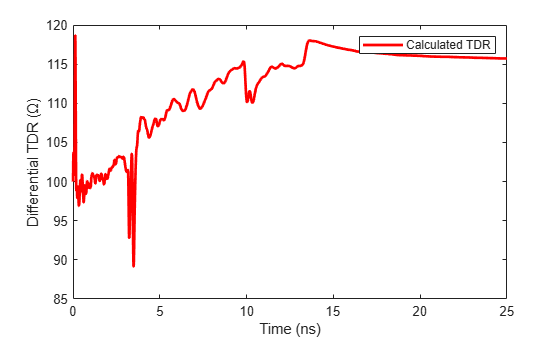# Compute Differential TDR and TDT

This example shows how to use RF Toolbox™ functions to calculate the time-domain reflectometry (TDR) and time-domain transmission (TDT) of a differential high-speed backplane channel.

You can use TDR and TDT waveforms to characterize high-speed channels, transmission lines, and other electrical systems.

### Read Single-Ended 4-Port S-Parameters and Convert Them to Differential 2-Port S-Parameters

Use the `sparameters` object to extract S-parameters from the `default.s4p`Touchstone file. The parameters in this data file are the 50-ohm S-parameters of a single-ended 4-port passive circuit, measured at 1496 frequencies ranging from 50 MHz to 15 GHz. Next, extract the single-ended four-port S-parameters from the data object.

```filename = 'default.s4p'; backplane = sparameters(filename); data = backplane.Parameters; freq = backplane.Frequencies; z0 = backplane.Impedance;```

Use the matrix conversion function `s2sdd` to convert the 4-port S-parameters to 2-port differential S-parameters.

```diffdata = s2sdd(data); diffsparams = sparameters(diffdata,freq,2*z0); z0differential = diffsparams.Impedance;```

### Calculate and Plot the Differential TDR

TDR is the time-domain reflected signal from any impedance discontinuities within a circuit. First, extract the differential S11 (reflected signal in the frequency domain) data using the `rfparam` function. Convert the S11 data to a time-domain signal by creating a rational function of the data using the `rational` function. Compute the TDR Signal using the `stepresp` function of the `rfmodel.rational` object.

```s11 = rfparam(diffsparams,1,1); s11fit = rational(freq,s11); Ts = 5e-12; N = 5000; Trise = 5e-11; [tdr,tdrT] = stepresp(s11fit,Ts,N,Trise);```

Calculate the impedance over time using the `gamma2z` function and plot the calculated TDR impedance versus time.

```zLt = gamma2z(tdr, z0differential); figure plot(tdrT*1e9,zLt,'r','LineWidth',2) ylabel('Differential TDR (Ω)') xlabel('Time (ns)') legend('Calculated TDR')```### Calculate and Plot Differential TDT

TDT is the time-domain transmitted voltage signal from an input in an electrical circuit. Use the `rational` function perform rational fitting on the TDT frequency data, then use the `stepresp` function to compute the TDT signal. Calculate the final TDT voltage signal by using the input voltage (S-parameters are normalized to 1 V by the equipment). Plot the calculated TDT.

```s21 = rfparam(diffsparams,2,1); s21fit= rational(freq,s21); Ts = 5e-12; N = 5000; Trise = 5e-11; [tdt,tdtT] = stepresp(s21fit, Ts, N, Trise); Vin = 1; tdtV = Vin*tdt/2; figure plot(tdtT*1e9,tdtV,'r','LineWidth',2) ylabel('Differential TDT (V)') xlabel('Time (ns)') legend('Calculated TDT')```### References

 Zeng. R, J. Sinsky. "Modified Rational Function Modeling Technique for High Speed Circuits." MTT-S Int. Microwave Symp. Dig,, San Fancisco, CA, June 11-16, 2006.

 A. S. Ali, R. Mittra. "Time-Domain Reflectometry using Scattering Parameters and a De-Embedding Application" Technical Report, Electromagnetic Communication Laboratory Report No. 86-4, May 1986.